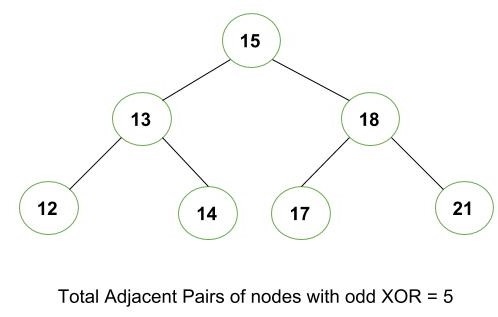# Count all pairs of adjacent nodes whose XOR is an odd number

Given a Binary Tree as shown below. The task is to count all pair of adjacent nodes whose XOR is an odd number.Explanation:

```Initially, root will be 0, start traversing the tree.
XOR of 15 and 13 will be  2 (Even)
XOR of 13 and 12 will be 1 (Odd)
XOR of 13 and 14 will be 5 (Even)
XOR of 15 and 18 will be 13 (Odd)
XOR of 18 and 17 will be 3 (Odd)
XOR of 18 and 21 will be 7 (Odd)

Therefore, total adjacent pairs with odd XOR = 5
```

## Recommended: Please try your approach on {IDE} first, before moving on to the solution.

Approach:

1. Start traversing the tree from top to down.
2. Every time perform XOR operation with the current node data and its adjacent data.
3. If XOR of both node is an odd number then increment the count.

Below is the implementation of the above approach:

## C++

 `// CPP program to find number of adjacent pair ` `// in Binary Tree with odd xor ` ` `  `#include ` `using` `namespace` `std; ` ` `  `// Tree Node ` `struct` `Node { ` `    ``int` `data; ` `    ``struct` `Node *left, *right; ` `}; ` ` `  `// Function to find number of adjacent pair ` `// in Binary Tree with odd xor ` `int` `countOddXor(Node* root, Node *parent=NULL) ` `{ ` `    ``// If Node is empty ` `    ``if` `(root == NULL) ` `        ``return` `0; ` ` `  `    ``// check pair of XOR is odd or not ` `    ``int` `res = 0; ` `    ``if` `(parent != NULL && (parent->data ^ root->data) % 2)  ` `        ``res++; ` ` `  `    ``return` `res + countOddXor(root->left, root) +  ` `                 ``countOddXor(root->right, root); ` `} ` ` `  `// Utility function to create a new tree node ` `Node* newNode(``int` `data) ` `{ ` `    ``Node* temp = ``new` `Node; ` `    ``temp->data = data; ` `    ``temp->left = NULL; ` `    ``temp->right = NULL; ` `    ``return` `temp; ` `} ` ` `  `// Driver code ` `int` `main() ` `{ ` `    ``struct` `Node* root = NULL; ` ` `  `    ``root = newNode(15); ` `    ``root->left = newNode(13); ` `    ``root->left->left = newNode(12); ` `    ``root->left->right = newNode(14); ` `    ``root->right = newNode(18); ` `    ``root->right->left = newNode(17); ` `    ``root->right->right = newNode(21); ` ` `  `    ``printf``(``"%d "``, countOddXor(root)); ` ` `  `    ``return` `0; ` `} `

## Java

 `// Java program to find number of adjacent pair ` `// in Binary Tree with odd xor ` `class` `GFG ` `{ ` ` `  `// Tree Node ` `static` `class` `Node ` `{ ` `    ``int` `data; ` `    ``Node left, right; ` `}; ` ` `  `// Function to find number of adjacent pair ` `// in Binary Tree with odd xor ` `static` `int` `countOddXor(Node root, Node parent) ` `{ ` `    ``// If Node is empty ` `    ``if` `(root == ``null``) ` `        ``return` `0``; ` ` `  `    ``// check pair of XOR is odd or not ` `    ``int` `res = ``0``; ` `    ``if` `(parent != ``null` `&&  ` `       ``(parent.data ^ root.data) % ``2` `== ``1``)  ` `        ``res++; ` ` `  `    ``return` `res + countOddXor(root.left, root) +  ` `                 ``countOddXor(root.right, root); ` `} ` ` `  `// Utility function to create a new tree node ` `static` `Node newNode(``int` `data) ` `{ ` `    ``Node temp = ``new` `Node(); ` `    ``temp.data = data; ` `    ``temp.left = ``null``; ` `    ``temp.right = ``null``; ` `    ``return` `temp; ` `} ` ` `  `// Driver code ` `public` `static` `void` `main(String[] args) ` `{ ` `    ``Node root = ``null``; ` ` `  `    ``root = newNode(``15``); ` `    ``root.left = newNode(``13``); ` `    ``root.left.left = newNode(``12``); ` `    ``root.left.right = newNode(``14``); ` `    ``root.right = newNode(``18``); ` `    ``root.right.left = newNode(``17``); ` `    ``root.right.right = newNode(``21``); ` ` `  `    ``System.out.printf(``"%d "``, countOddXor(root, ``null``)); ` `} ` `} ` ` `  `// This code is contributed by PrinciRaj1992 `

## C#

 `// C# program to find number of adjacent pair ` `// in Binary Tree with odd xor ` `using` `System; ` ` `  `class` `GFG ` `{ ` ` `  `// Tree Node ` `public` `class` `Node ` `{ ` `    ``public` `int` `data; ` `    ``public` `Node left, right; ` `}; ` ` `  `// Function to find number of adjacent pair ` `// in Binary Tree with odd xor ` `static` `int` `countOddXor(Node root,  ` `                       ``Node parent) ` `{ ` `    ``// If Node is empty ` `    ``if` `(root == ``null``) ` `        ``return` `0; ` ` `  `    ``// check pair of XOR is odd or not ` `    ``int` `res = 0; ` `    ``if` `(parent != ``null` `&&  ` `       ``(parent.data ^ root.data) % 2 == 1)  ` `        ``res++; ` ` `  `    ``return` `res + countOddXor(root.left, root) +  ` `                 ``countOddXor(root.right, root); ` `} ` ` `  `// Utility function to create a new tree node ` `static` `Node newNode(``int` `data) ` `{ ` `    ``Node temp = ``new` `Node(); ` `    ``temp.data = data; ` `    ``temp.left = ``null``; ` `    ``temp.right = ``null``; ` `    ``return` `temp; ` `} ` ` `  `// Driver code ` `public` `static` `void` `Main(String[] args) ` `{ ` `    ``Node root = ``null``; ` ` `  `    ``root = newNode(15); ` `    ``root.left = newNode(13); ` `    ``root.left.left = newNode(12); ` `    ``root.left.right = newNode(14); ` `    ``root.right = newNode(18); ` `    ``root.right.left = newNode(17); ` `    ``root.right.right = newNode(21); ` ` `  `    ``Console.WriteLine(``"{0} "``,  ` `          ``countOddXor(root, ``null``)); ` `} ` `} ` ` `  `// This code is contributed by 29AjayKumar `

Output:

```5
```

My Personal Notes arrow_drop_upCheck out this Author's contributed articles.

If you like GeeksforGeeks and would like to contribute, you can also write an article using contribute.geeksforgeeks.org or mail your article to contribute@geeksforgeeks.org. See your article appearing on the GeeksforGeeks main page and help other Geeks.

Please Improve this article if you find anything incorrect by clicking on the "Improve Article" button below.

Improved By : princiraj1992, 29AjayKumar

Article Tags :
Practice Tags :

1

Please write to us at contribute@geeksforgeeks.org to report any issue with the above content.